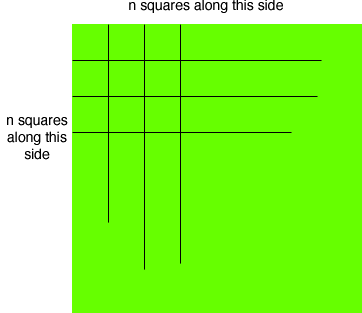Quandaries and Queries I know that there are 27,878,400 sq ft in a square mile and that there are 640 acres in a sq mile. But how do I figure out the measurement of each acre. If I could make every acre a square what would it's measurement be? Thanks Hi Rudy, If you are thinking of taking a plot of land that is one mile by one mile and dividing it into squares, each of size one acre you're going to find that you can't do it. The difficulty arises because you need 640 such squares. Suppose that you start by marking out n squares along the mile long north side. You then see that you need the same number n of squares along the west side.Hence, you have nn = n2, one acres squares. But there is no integer n so that n2 is 640. The closest you can get in n being 25 or 26. 252 = 625 and 262 = 676. Penny